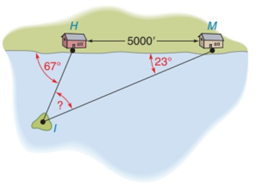Chapter 2.4, Problem 35E### Elementary Geometry for College St...

6th Edition
Daniel C. Alexander + 1 other
ISBN: 9781285195698

#### Solutions

Chapter
Section### Elementary Geometry for College St...

6th Edition
Daniel C. Alexander + 1 other
ISBN: 9781285195698
Textbook Problem
15 views

# Along a straight shoreline, two houses are located at points H and M . The houses are 5000 feet apart. A small island lies in view of both houses, with angles as indicated. Find m ∠ I .To determine

To find:

The value of mI.

Explanation

Given:

The houses are 5000 feet apart.

Figure (1)

Approach:

The exterior angle of a triangle is equal to sum of the measure of two non-adjacent interior angles.

Calculation:

In triangle ΔIHM, the exterior angle is the sum of the measure of angle mI and 67°.

Since, the exterior angle is 67°

### Still sussing out bartleby?

Check out a sample textbook solution.

See a sample solution

#### The Solution to Your Study Problems

Bartleby provides explanations to thousands of textbook problems written by our experts, many with advanced degrees!

Get Started

#### Define sampling with replacement and explain why is it used?

Statistics for The Behavioral Sciences (MindTap Course List)

#### Solve for x: e2x1 = 10. a) 12(1+10e) b) 3 + ln 10 c) 2 + ln 10 d) 1+ln102

Study Guide for Stewart's Single Variable Calculus: Early Transcendentals, 8th

#### Find for x = 3t2 + 1, y = t6 + 6t5. t4 + 5t3 4t3 + 15t2

Study Guide for Stewart's Multivariable Calculus, 8th

#### What is the primary factor that determines when it is time to change phases?

Research Methods for the Behavioral Sciences (MindTap Course List)